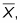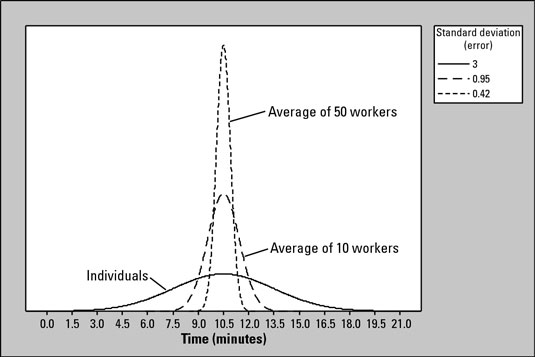##### Statistics For DummiesIn statistics, when the original distribution for a population X is normal, then you can also assume that the shape of the sampling distribution, orwill also be normal, regardless of the sample size n.

For example, if you look at the amount of time (X) required for a clerical worker to complete a task, you may find that X had a normal distribution (refer to the lowest curve in the figure).Distributions of times for 1 worker, 10 workers, and 50 workers.

If you refer to the other curves in the figure, you see the average times for samples of n = 10 and n = 50 clerical workers, respectively, also have normal distributions.

When X has a normal distribution, the sample means also always have a normal distribution, no matter what size samples you take, even if you take samples of only 2 clerical workers at a time to calculate the sample mean.

The difference between the curves in the figure is not their means or their shapes, but rather their amount of variability (how close the values in the distribution are to the mean). Results based on large samples vary less and will be more concentrated around the mean than results from small samples or results from the individuals in the population.